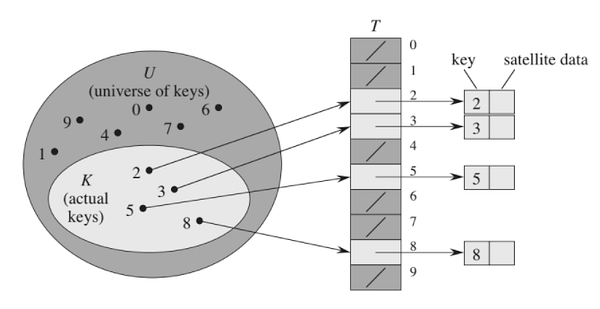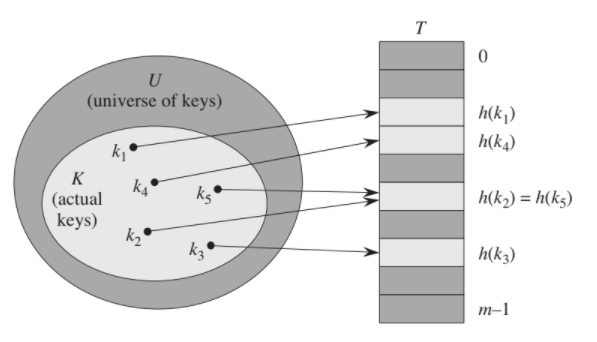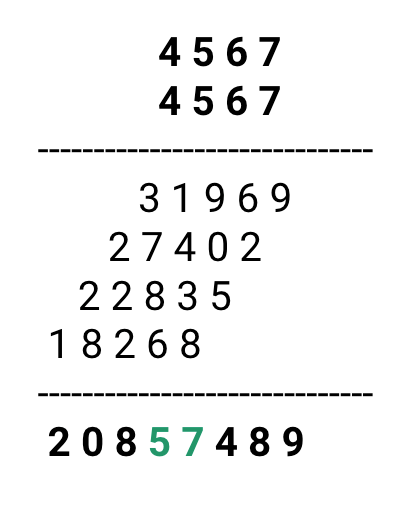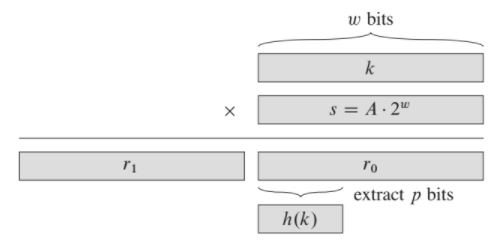# Introduction to Hashing

Millions of users on the internet are generating terabytes of content every day, and the amazing part is: the amount of content is doubling every six months! So we need an effcient search mechanism or new data structures for storing and accessing data with such growth. From another perspective, Many applications require a mechanism that supports only the dictionary operations: Insert, Search, and Delete. For example, a compiler that translates a programming language maintains a symbol table, in which the keys of elements are character strings corresponding to identifiers in the language.

But the critical question is: what is wrong with the traditional data structures like arrays and linked lists? If the large data is stored in an array, the amount of time required to search an element in the array is either O(log n) or O(n) based on whether the array is sorted or not. At least the O(n) scenario may not be a viable option if we need to process a huge data set. On another side, searching for an element in a hash table can take O(n) time in the worst case, but in practice, hashing performs exceptionally well. Under reasonable assumptions, the average time to search for an element in a hash table is O(1). So, it's time to deep dive to understand the efficient idea of hashing and hash tables!

Direct addressing is a basic idea of hashing that works well when all possible keys are small in number. Suppose an application requires a data storage mechanism in which each element has a key that is not too large. We can assume that no two elements have the same key i.e. keys are distinct and the range of keys is 0 to m-1. For the implementation, we can set up an array T[0..m-1] in which:

• T[i] = k, if key of data k = i
• T[i] = NULL, otherwise

This is a direct address table where each dictionary operation (Insert, Search, and Delete) will take O(1) time! But what is the problem here? Think!Image reference: CLRS Book

In the above image, each key in the universe U = {0, 1, 2,....9} corresponds to an index in the table. The set K = {2, 3, 5, 8} of actual keys determines the slots in the table that contain pointers to elements. For some applications, the direct-address table itself can hold the elements in the memory: Rather than storing values of a key with a pointer from a slot in the table, we can save the space by storing the value in the slot itself. However, we must have some way to tell whether the slot is empty or not. Think!

### The Idea of the Hash Table

Direct addressing examines an arbitrary position in an array in O(1) time, but we can only take advantage of it when we can afford an array that has one slot for every possible key. When the actual number of keys is small compared to the total number of possible keys, a hash table is an effective alternative to a direct address table. In other words, a hash table uses an array of size proportional to the number of actual keys and calculates the array index from the key rather than using the key as an array index directly. So the idea of the hash table generalizes the concept of a normal array.

In hashing, the key does not have to be an integer. We can use the name as a key or use some other relevant information of the input data. The idea is to choose a good hash function and compute an index to store data at a specific slot in a table. If keys are small integers, we can use the direct addressing method by interpreting the key as an array index for immediate access.So the downside of direct addressing is obvious: if the universe of all possible keys is large, storing a table T of size equal to all possible keys may be impractical or even impossible, given the memory available on a typical computer. Furthermore, the set K of keys stored may be so small, and most of the space allocated would be wasted.

When the set K of keys stored is much smaller than the universe of all possible keys, a hash table requires much less storage than a direct-address table. Specifically, we can reduce the storage requirement to O(m) (where m is the total number of actual keys)while maintaining the benefit that is searching for an element in the hash table still requires only O(1) time on average.

We use a hash function h to compute the slot from the key k and the store element at slot h(k) in a hash table. In other words, we can say that an element with key k hashes to slot h(k), which is the hash value of key k. So hash function reduces the range of array indices and hence the size of the table.

There is one hitch: two keys may hash to the same slot. We call this situation a collision. Fortunately, we have effective techniques for resolving the conflict created by collisions. Of course, the ideal solution would be to avoid collisions altogether. We might try to achieve this goal by choosing a suitable hash function. Thus, we need a well-designed “random” looking hash function to minimize the number of collisions, but we still need a method for resolving the collisions. In a separate blog, we will discuss two different approaches to collision resolution: chaining and open addressing.

So we store (key, value) pairs in an array by transforming keys into array indices using a hash function. Searching using hashing consists of three separate parts:

• Pre-processing to calculate the search key(a natural number) from the given input data.
• Using a hash function to transform the search key into an array index.
• Inserting or accessing the desired value using a key.

The mathematical perspective of the Hash Table

The map data structure, in a mathematical sense, is a relation between two sets. We can define a map M as a set of pairs, where each pair is in the form (key, value), where for a given key, we can find a value using some function that map keys to values. Most simply, we can think of an array as a map where the key is the index, and the value is the value available at that index i.e. for a given array A[] if i is the key, then we can find the value by simply looking up A[i].

If there were no memory limitations, we could search with only one memory access by simply using the key as an index in a potentially large array. This ideal situation often cannot be achieved because the amount of memory required is not a practical choice when the total number of possible keys is larger than the actual number of keys. On the other hand, we can use the linear search in an unordered array if there were no time limitations.

So hashing provides a way to use a reasonable amount of memory and time to balance these two extremes. Indeed, it turns out that we can trade off time and memory in hashing algorithms by adjusting parameters, not by rewriting code! Think!

### Introduction to Hash Functions

If we have an array that can store m key-value pairs, then we need a hash function to transform any given key into an index into that array: an integer in the range [0, m - 1]. Here we need a hash function that is easy to compute and uniformly distributes the keys i.e. for each key, every integer between 0 and m-1 should be equally likely.

In other words, a good hash function satisfies (approximately) the assumption of uniform hashing: each key is equally likely to hash to any of the m slots. Unfortunately, this ideal situation is challenging to achieve, which makes the understanding of hashing interesting! Critical questions are: How do we implement a hash function which is close to the ideal situation? Think!

If the function is easy to compute, then accessing the key is also easy. However, since all possible keys are large and the table is small, no matter what function we use, many keys will be mapped into the same place in the table. So we need to deal with two situations: (1) finding a hash function that minimizes the likelihood of collisions, and (2) handling collisions.

Even though all possible keys are larger than the size of the table, the actual set of keys we handle is usually not too large. Of course, no hash function can map all possible sets of keys without collisions. If the mapping is uniform, If the number of keys is n and the size of the hash table is m, then each location will have approximately n/m keys mapped into it.

A basic hash function

If the keys are real numbers between 0 and 1, we can just multiply each key by m and round off to the nearest integer to get an index between 0 and m-1 i.e. the hash function is h(k) = ⌊km⌋

Although this approach looks good, it is defective because it gives more weight to the most significant bits of the keys and the least significant bits play no role. One way to address this situation is to use modular hashing on the binary representation of the key. Think!

The division method or modular hashing

This is the most commonly used method for hashing which is also called modular hashing. In the division method of hash functions, we map a key k into one of the m slots by taking the remainder of k divided by m i.e. the hash function is h(k) = k mod m. For example, if the hash table has size m = 12 and the key is k = 100, then h(k) = 4. Since it requires only a single division operation, hashing by division is quite fast. But when using the division method, we usually avoid specific values of m or choose a specific value of m:

1. m should not be a power of 2, since if m = 2^p, then h(k) is just the p lowest-order bits of k. Unless we know that all low-order p-bit patterns are equally likely, we are better off designing the hash function to depend on all the bits of the key.
2. We should choose the array size m to be prime and, for any positive integer key k. This approach is effective in dispersing the keys evenly between 0 and m  -  1 and minimize the collision of keys. If m is not prime, it may be the case that not all of the bits of the key play a role and the hash function might not disperse the values evenly. A prime not too close to an exact power of 2 is often a good choice for m.

• Suppose if the keys are base-10 numbers and M is 10^k, then only the k least significant digits are used. Such a choice might be problematic!
• Suppose we wish to allocate a hash table, with collisions resolved by chaining, to hold roughly n = 2000 keys. We don’t mind examining an average of 3 elements in an unsuccessful search, so we allocate a hash table of size m = 701. We could choose m = 701 because it is a prime near 2000/3 but not near any power of 2. Treating each key k as an integer, our hash function would be h(k) = k mod 701.
• Suppose that the keys are three-digit telephone area codes and m = 100. If the middle digit of area codes is 0 or 1, so this choice strongly favors the values less than 20. A better idea would be to use the prime value to better disperses them (a prime value not close to 100 would work better). Similarly, IP addresses that are used on the internet are binary numbers that are not random for similar reasons, so we need to use a table size that is a prime (in particular, not a power of 2) if we want to use modular hashing to disperse them.
3. If the size of the table cannot be adjusted easily to be a prime, then the following hash function can be used: h(k) =(k mod p) mod m, where p is a prime, and p > m (p should be sufficiently larger than m to be effective, but it should also be sufficiently smaller than the number of all possible values of keys). Another fact is: Finding a large list of primes, however, is not easy. Another possibility is the following: At random, select two numbers a and b, such that a,b <p, and a *0, and let h(k) = (ak + b mod p) mod m. This function is more complicated to compute than the previous one is, but it has the advantage that it is very good on average for all inputs.
4. As we have already mentioned, no hash function can be good for all inputs. Using primes as described is fairly safe since most data in practice have no structure related to prime numbers. On the other hand, it is always possible (although unlikely) that, in a certain application, one will want to store results of some experiments made on integers all of which are of the form r + kp for a constant r. All these numbers of course will have the same hash values if m = p is used. We can take the idea of scrambling data with hashing one step further, and use a random procedure to select a hash function!
5. Here is another example! if there are 250 students identified by their social security number, we will not allocate an array of size 1 billion to store information about them (there are 1 billion possible social security numbers). Instead, we can use the last three digits of the numbers, in which case we need only an array of size 1000. But there may be students with the same last three digits (in fact, with 250 students, the probability of that is quite high). We can also use the last four digits, or the last three digits and the first letter of the student’s name, to minimize duplicates even further. However, using more digits requires a larger-size table and results in a smaller utilization.

A simple Hash Function for strings

Most of the hash functions assume that the keys are a set of natural numbers, i.e., N = {0, 1, 2, 3, ....}. Thus, if the keys are not natural numbers, we need to find a way to interpret them as natural numbers. For example, we can interpret a string as the sum of the ASCII values of the characters in a string. We can also design other methods for converting each key as a large natural number in a given application.

``````int stringHashFunction(String s[], int size, int m)
{
int sum = 0
for(int i = 0; i < size; i = i + 1)
sum = sum + s[i]
return sum % m
}
``````

String folding: a better hash function for strings

This function takes a string as input and processes the string four bytes simultaneously and interprets each of the four-byte chunks as a single long integer value. The integer values for the four-byte chunks are added together and the resulting sum is converted to the range 0 to m−1 using the modulus operator.

``````int stringFolding(String s[], int size, int m)
{
long sum = 0
long mul = 1
for (int i = 0; i < size; i = i + 1)
{
mul = (i % 4 == 0) ? 1 : mul * 256
sum = sum + s[i] * mul
}
return (int)(sum % m)
}
``````

For example, if the string “aaaabbbb” is given, then the first four bytes (“aaaa”) will be interpreted as the integer value 1,633,771,873, and the next four bytes (“bbbb”) will be interpreted as the integer value 1,650,614,882. Their sum is 3,284,386,755 (when treated as an unsigned integer). If the table size is 101 then the modulus function will cause this key to hash to slot 75 in the table.

The Mid-Square method

A good hash function to use with integer key values is the mid-square method. The mid-square method squares the key value and then takes out the middle r bits of the result, giving a value in the range 0 to 2^r - 1. This works well because most or all bits of the key-value contribute to the result.

For example, consider records whose keys are 4-digit numbers in base 10. The goal is to hash these key values to a table of size 100 i.e. a range of 0 to 99. This range is equivalent to two digits in base 10, so r = 2. If the input is the number 4567, squaring yields an 8-digit number, 20857489.The middle two digits of this result are 57. All digits of the original key value (equivalently, all bits when the number is viewed in binary) contribute to the middle two digits of the squared value. Thus, the result is not dominated by the distribution of the bottom digit or the top digit of the original key value. Of course, if the key values all tend to be small numbers, then their squares will only affect the low-order digits of the hash value.

The multiplication method The multiplication method for creating hash functions operates in two steps. First, we multiply the key k by a constant A in the range 0< A < 1 and extract the fractional part of kA. Then, we multiply this value by m and take the floor of the result. In short, the hash function is h(k) = ⌊m(kA mod 1)⌋, where “kA mod 1” means the fractional part of kA, that is, kA - ⌊kA⌋.An advantage of the multiplication method is that the value of m is not critical. We typically choose it to be a power of 2 (m = 2^p for some integer p) since we can easily implement the function on most computers. Suppose that the machine's word size is w bits and that k fits into a single word. We restrict A to be a fraction of the form s = 2w, where s is an integer in the range 0 < s < 2w.

We first multiply k by the w-bit integer s = A*2^w. The result is a 2w-bit value r12^w + r0, where r1 is the high-order word of the product and r0 is the low-order word of the product. The desired p-bit hash value consists of the p most significant bits of r0. Although this method works with any value of the constant A, it works better with some values than others. The optimal choice depends on the characteristics of the data being hashed. Think!

### Special note around hash functions

• Hash functions should transform a set of keys uniformly to a set of random locations in the range 1 to m. Uniformity and randomness are the essences of hashing. For example, instead of taking the last three digits of the social security number, we could take the last three digits of the student’s year of birth. It is clear that this is an inferior hash function since it is much more likely that many students were born in the same year than it is that many students have the same last three digits of the social security number.
• A good hash function generates the hash values from keys in a way independent of any patterns that might exist in the data. For example, the modular hashing frequently gives good results, assuming that we choose m as a prime number that is unrelated to any patterns in the distribution of keys.
• Of course, the same hash function must be used for all accesses to the same table. In many cases, however, there is a need for many independent tables or tables that are created and destroyed frequently. In those cases, a different hash function can be used every time a different table is created. The random hash functions described above have certain other desirable properties.
• Information about the distribution of keys can help us to design an effcient hash function. For example, suppose the keys are strings representing the name of the person, then closely related names, such as "Mohan", "Sohan", "Rohan" may often occur. Here a good hash function would minimize the chance that such names hash to the same slot in the hash table. In other words, some applications of hash functions might require stronger properties than the condition of the simple uniform hashing. For example, we might want keys that are “close” to yield hash values that are far apart.

In the next series of blogs, we will discuss collision resolutions techniques, implementation of hash table operations, and problem-solving using hashing. Enjoy learning, Enjoy thinking, Enjoy algorithms!

### References

• Book: Algorithms by CLRS
• Book: Algorithms by Robert Sedgewick
• Lectures of Introduction to Algorithms by MIT OpenCourseWare
• Book: Algorithm design manual by Steven Skiena

Get well-designed application and interview centirc content on ds-algorithms, machine learning, system design and oops. Content will be delivered weekly.